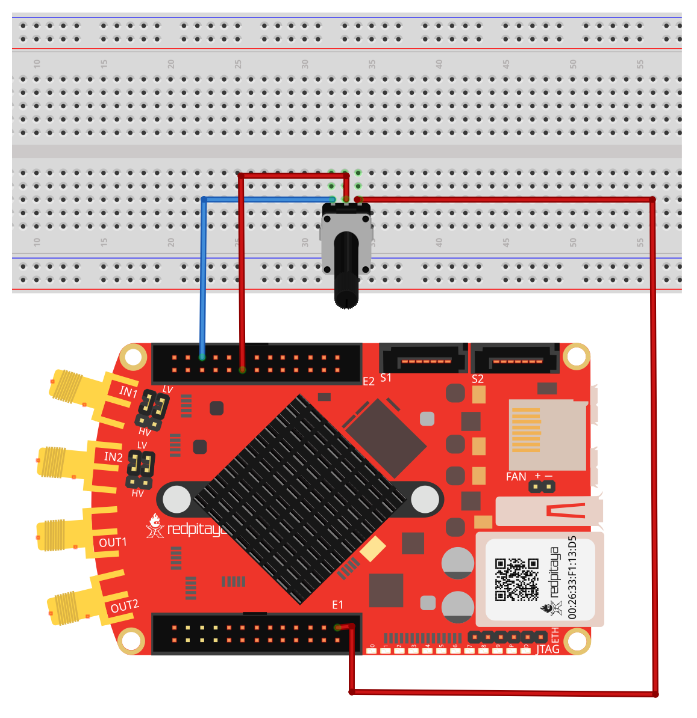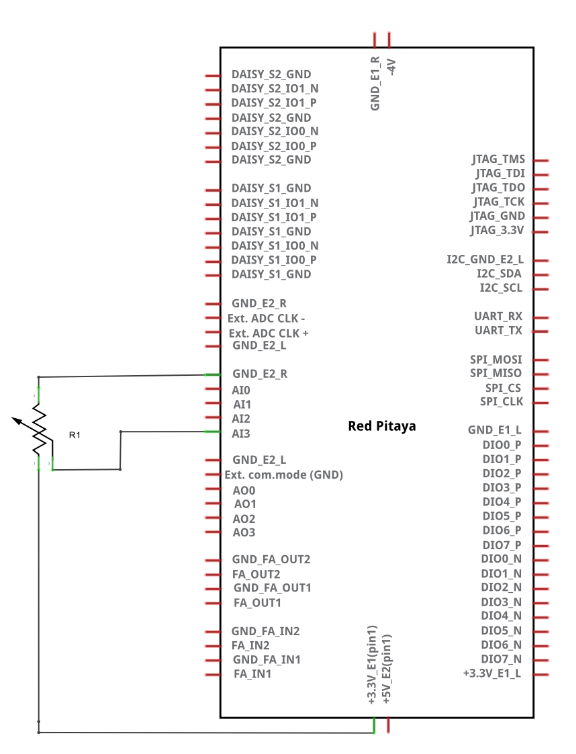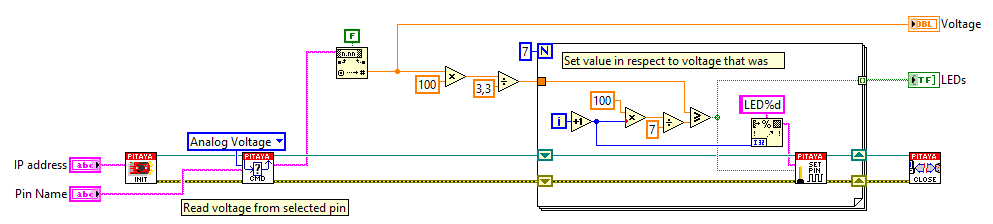# 2.3.1.4.2.1. Read analog voltage on a slow analog input¶

## 2.3.1.4.2.1.1. Description¶

This example shows how to measure the analog voltage of slow analog inputs on the Red Pitaya extension connector. The analog inputs on the Red Pitaya are rated from 0 to 3.3 Volts.

## 2.3.1.4.2.1.2. Required hardware¶

• Red Pitaya device

• R1 10k potentiometer

Wiring example for STEMlab 125-14 & STEMlab 125-10:## 2.3.1.4.2.1.3. Circuit¶## 2.3.1.4.2.1.4. Code - MATLAB®¶

The code is written in MATLAB. In the code, we use SCPI commands and TCP client communication. Copy the code from below into the MATLAB editor, save the project, and hit the “Run” button.

```%% Define Red Pitaya as TCP client object

IP = '192.168.178.108';           % Input IP of your Red Pitaya...
port = 5000;
RP = tcpclient(IP, port);

%% Open connection with your Red Pitaya

RP.ByteOrder = "big-endian";
configureTerminator(RP,"CR/LF");

volts0 = str2double(writeread(RP,'ANALOG:PIN? AIN0'));
volts1 = str2double(writeread(RP,'ANALOG:PIN? AIN1'));
volts2 = str2double(writeread(RP,'ANALOG:PIN? AIN2'));
volts3 = str2double(writeread(RP,'ANALOG:PIN? AIN3'));

%% Close connection with Red Pitaya

clear RP;
```

## 2.3.1.4.2.1.5. Code - C¶

Note

Although the C code examples don’t require the use of the SCPI server, we have included them here to demonstrate how the same functionality can be achieved with different programming languages. Instructions on how to compile the code are here.

```/* Read analog voltage on slow analog input */

#include <stdio.h>
#include <stdlib.h>

#include "rp.h"

int main (int argc, char **argv) {
float value ;

// Initialization of API
if (rp_Init() != RP_OK) {
fprintf(stderr, "Red Pitaya API init failed!\n");
return EXIT_FAILURE;
}

// Measure each XADC input voltage
for (int i=0; i<4; i++) {
rp_AIpinGetValue(i, &value[i]);
printf("Measured voltage on AI[%i] = %1.2fV\n", i, value[i]);
}

// Releasing resources
rp_Release();

return EXIT_SUCCESS;
}
```

## 2.3.1.4.2.1.6. Code - Python¶

```import sys
import redpitaya_scpi as scpi

rp_s = scpi.scpi(sys.argv)

for i in range(4):
rp_s.tx_txt('ANALOG:PIN? AIN' + str(i))
value = float(rp_s.rx_txt())
print ("Measured voltage on AI["+str(i)+"] = "+str(value)+"V")
```

## 2.3.1.4.2.1.7. Code - Scilab¶

How to set sockets is described in the Blink example.

```clc

// Load SOCKET Toolbox
SOCKET_init();

// Define Red Pitaya as TCP/IP object

IP= '192.168.178.56';            // Input IP of your Red Pitaya...
port = 5000;                     // If you are using WiFi then IP is:
tcpipObj='RedPitaya';            // 192.168.128.1

// Open connection with your Red Pitaya

SOCKET_open(tcpipObj,IP,port);

// Red value on analog input 3

volts=strtod(SOCKET_query(tcpipObj,'ANALOG:PIN? AIN3'));
disp(volts)

// Define value p from 0 - 100 //

p = volts *(100/3.3) ;   // Set value of p in respect to readed voltage

if p >=(100/7)
SOCKET_write(tcpipObj,'DIG:PIN LED1,1')
else
SOCKET_write(tcpipObj,'DIG:PIN LED1,0')
end

if p >=(100/7)*2
SOCKET_write(tcpipObj,'DIG:PIN LED2,1')
else
SOCKET_write(tcpipObj,'DIG:PIN LED2,0')
end

if p >=(100/7)*3
SOCKET_write(tcpipObj,'DIG:PIN LED3,1')
else
SOCKET_write(tcpipObj,'DIG:PIN LED3,0')
end

if p >=(100/7)*4
SOCKET_write(tcpipObj,'DIG:PIN LED4,1')
else
SOCKET_write(tcpipObj,'DIG:PIN LED4,0')
end

if p >=(100/7)*5
SOCKET_write(tcpipObj,'DIG:PIN LED5,1')
else
SOCKET_write(tcpipObj,'DIG:PIN LED5,0')
end

if p >=(100/7)*6
SOCKET_write(tcpipObj,'DIG:PIN LED6,1')
else
SOCKET_write(tcpipObj,'DIG:PIN LED6,0')
end

if p >=(100/7)*7
SOCKET_write(tcpipObj,'DIG:PIN LED7,1')
else
SOCKET_write(tcpipObj,'DIG:PIN LED7,0')
end

// Close connection with Red Pitaya

SOCKET_close(tcpipObj);
```

## 2.3.1.4.2.1.8. Code - LabVIEW¶